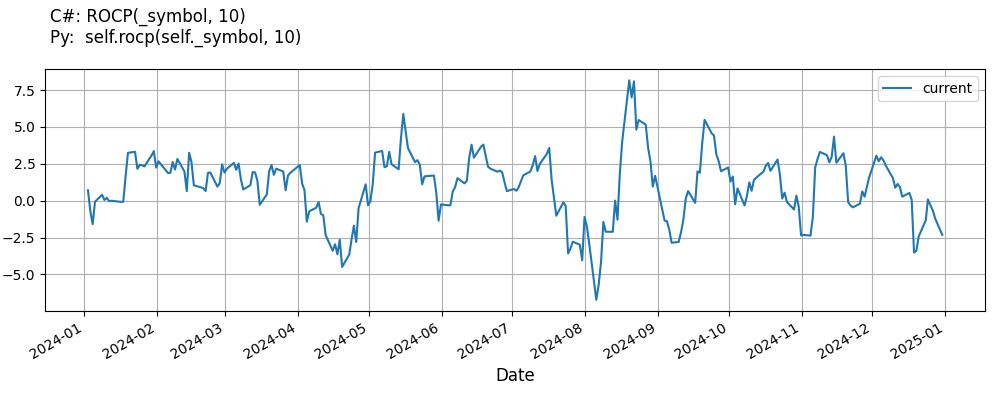# Supported Indicators

## Rate Of Change Percent

### Introduction

This indicator computes the n-period percentage rate of change in a value using the following: 100 * (value_0 - value_n) / value_n

To view the implementation of this indicator, see the LEAN GitHub repository.

### Using ROCP Indicator

To create an automatic indicators for RateOfChangePercent, call the ROCP helper method from the QCAlgorithm class. The ROCP method creates a RateOfChangePercent object, hooks it up for automatic updates, and returns it so you can used it in your algorithm. In most cases, you should call the helper method in the Initialize method.

public class RateOfChangePercentAlgorithm : QCAlgorithm
{
private Symbol _symbol;
private RateOfChangePercent _rocp;

public override void Initialize()
{
_rocp = ROCP(_symbol, 10);
}

public override void OnData(Slice data)
{
{
// The current value of _rocp is represented by itself (_rocp)
// or _rocp.Current.Value
Plot("RateOfChangePercent", "rocp", _rocp);

}
}
}
class RateOfChangePercentAlgorithm(QCAlgorithm):
def Initialize(self) -> None:
self.rocp = self.ROCP(self.symbol, 10)

def OnData(self, slice: Slice) -> None:
# The current value of self.rocp is represented by self.rocp.Current.Value
self.Plot("RateOfChangePercent", "rocp", self.rocp.Current.Value)



The following reference table describes the ROCP method:

### ROCP()1/1

            RateOfChangePercent QuantConnect.Algorithm.QCAlgorithm.ROCP (
Symbol                           symbol,
Int32                            period,
*Nullable<Resolution>      resolution,
*Func<IBaseData, Decimal>  selector
)


Creates a new RateOfChangePercent indicator. This will compute the n-period percentage rate of change in the security. The indicator will be automatically updated on the given resolution.

If you don't provide a resolution, it defaults to the security resolution. If you provide a resolution, it must be greater than or equal to the resolution of the security. For instance, if you subscribe to hourly data for a security, you should update its indicator with data that spans 1 hour or longer.

You can manually create a RateOfChangePercent indicator, so it doesn't automatically update. Manual indicators let you update their values with any data you choose.

Updating your indicator manually enables you to control when the indicator is updated and what data you use to update it. To manually update the indicator, call the Update method with time/number pair, or an IndicatorDataPoint. The indicator will only be ready after you prime it with enough data.

public class RateOfChangePercentAlgorithm : QCAlgorithm
{
private Symbol _symbol;
private RateOfChangePercent _rocp;

public override void Initialize()
{
_rocp = new RateOfChangePercent(10);
}

public override void OnData(Slice data)
{
if (data.Bars.TryGeValue(_symbol, out var bar))
{
_rocp.Update(bar.EndTime, bar.Close);
}

{
// The current value of _rocp is represented by itself (_rocp)
// or _rocp.Current.Value
Plot("RateOfChangePercent", "rocp", _rocp);

}
}
}
class RateOfChangePercentAlgorithm(QCAlgorithm):
def Initialize(self) -> None:
self.rocp = RateOfChangePercent(10)

def OnData(self, slice: Slice) -> None:
bar = slice.Bars.get(self.symbol)
if bar:
self.rocp.Update(bar.EndTime, bar.Close)

# The current value of self.rocp is represented by self.rocp.Current.Value
self.Plot("RateOfChangePercent", "rocp", self.rocp.Current.Value)



To register a manual indicator for automatic updates with the security data, call the RegisterIndicator method.

public class RateOfChangePercentAlgorithm : QCAlgorithm
{
private Symbol _symbol;
private RateOfChangePercent _rocp;

public override void Initialize()
{
_rocp = new RateOfChangePercent(10);
RegisterIndicator(_symbol, _rocp, Resolution.Daily);
}

public override void OnData(Slice data)
{
{
// The current value of _rocp is represented by itself (_rocp)
// or _rocp.Current.Value
Plot("RateOfChangePercent", "rocp", _rocp);

}
}
}
class RateOfChangePercentAlgorithm(QCAlgorithm):
def Initialize(self) -> None:
self.rocp = RateOfChangePercent(10)
self.RegisterIndicator(self.symbol, self.rocp, Resolution.Daily)

def OnData(self, slice: Slice) -> None:
# The current value of self.rocp is represented by self.rocp.Current.Value
self.Plot("RateOfChangePercent", "rocp", self.rocp.Current.Value)



The following reference table describes the RateOfChangePercent constructor:

### RateOfChangePercent()1/2

            RateOfChangePercent QuantConnect.Indicators.RateOfChangePercent (
int  period
)


Creates a new RateOfChangePercent indicator with the specified period.

### RateOfChangePercent()2/2

            RateOfChangePercent QuantConnect.Indicators.RateOfChangePercent (
string  name,
int     period
)


Creates a new RateOfChangePercent indicator with the specified period.

### Visualization

The following image shows plot values of selected properties of RateOfChangePercent using the plotly library.You can also see our Videos. You can also get in touch with us via Discord.